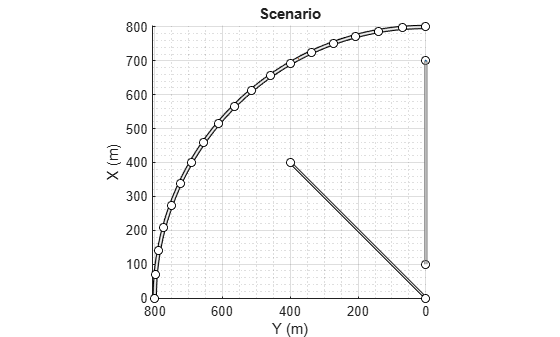# actorProfiles

Physical and radar characteristics of actors in driving scenario

## Syntax

``profiles = actorProfiles(scenario)``

## Description

example

````profiles = actorProfiles(scenario)` returns the physical and radar characteristics, `profiles`, for all actors in a driving scenario, `scenario`. Actors include `Actor` objects, `Vehicle` objects and `Barrier` segments, which you can create using the `actor`, `vehicle` and `barrier` functions, respectively.You can use actor profiles as inputs to radar, vision, and lidar sensors, such as `drivingRadarDataGenerator`, `visionDetectionGenerator` and `lidarPointCloudGenerator` objects.```

## Examples

collapse all

Create a driving scenario containing a curved road, two straight roads, and two actors: a car and a bicycle. Both actors move along the road for 60 seconds.

Create the driving scenario object.

`scenario = drivingScenario('SampleTime',0.1','StopTime',60);`

Create the curved road using road center points following the arc of a circle with an 800-meter radius. The arc starts at 0°, ends at 90°, and is sampled at 5° increments.

```angs = [0:5:90]'; R = 800; roadcenters = R*[cosd(angs) sind(angs) zeros(size(angs))]; roadwidth = 10; road(scenario,roadcenters,roadwidth);```

```roadcenters = [700 0 0; 100 0 0]; road(scenario,roadcenters)```
```ans = Road with properties: Name: "" RoadID: 2 RoadCenters: [2x3 double] RoadWidth: 6 BankAngle: [2x1 double] ```
```roadcenters = [400 400 0; 0 0 0]; road(scenario,roadcenters)```
```ans = Road with properties: Name: "" RoadID: 3 RoadCenters: [2x3 double] RoadWidth: 6 BankAngle: [2x1 double] ```

`rbdry = roadBoundaries(scenario);`

Add a car and a bicycle to the scenario. Position the car at the beginning of the first straight road.

```car = vehicle(scenario,'ClassID',1,'Position',[700 0 0], ... 'Length',3,'Width',2,'Height',1.6);```

Position the bicycle farther down the road.

```bicycle = actor(scenario,'ClassID',3,'Position',[706 376 0]', ... 'Length',2,'Width',0.45,'Height',1.5);```

Plot the scenario.

```plot(scenario,'Centerline','on','RoadCenters','on'); title('Scenario');```Display the actor poses and profiles.

`poses = actorPoses(scenario)`
```poses=2×1 struct array with fields: ActorID Position Velocity Roll Pitch Yaw AngularVelocity ```
`profiles = actorProfiles(scenario)`
```profiles=2×1 struct array with fields: ActorID ClassID Length Width Height OriginOffset MeshVertices MeshFaces RCSPattern RCSAzimuthAngles RCSElevationAngles ```

## Input Arguments

collapse all

Driving scenario, specified as a `drivingScenario` object.

## Output Arguments

collapse all

Actor profiles, returned as a structure or as an array of structures. Each structure contains the physical and radar characteristics of an actor.

The actor profile structures have these fields.

FieldDescription
`ActorID`Scenario-defined actor identifier, specified as a positive integer.
`ClassID`Classification identifier, specified as a nonnegative integer. `0` represents an object of an unknown or unassigned class.
`Length`Length of actor, specified as a positive real-valued scalar. Units are in meters.
`Width`Width of actor, specified as a positive real-valued scalar. Units are in meters.
`Height`Height of actor, specified as a positive real-valued scalar. Units are in meters.
`OriginOffset`Offset of actor's rotational center from its geometric center, specified as a real-valued vector of the form [x, y, z]. The rotational center, or origin, is located at the bottom center of the actor. For vehicles, the rotational center is the point on the ground beneath the center of the rear axle. Units are in meters.
`MeshVertices`Mesh vertices of actor, specified as an n-by-3 real-valued matrix of vertices. Each row in the matrix defines a point in 3-D space.
`MeshFaces`Mesh faces of actor, specified as an m-by-3 matrix of integers. Each row of `MeshFaces` represents a triangle defined by the vertex IDs, which are the row numbers of vertices.
`RCSPattern`Radar cross-section (RCS) pattern of actor, specified as a `numel(RCSElevationAngles)`-by-`numel(RCSAzimuthAngles)` real-valued matrix. Units are in decibels per square meter.
`RCSAzimuthAngles`Azimuth angles corresponding to rows of `RCSPattern`, specified as a vector of values in the range [–180, 180]. Units are in degrees.
`RCSElevationAngles`Elevation angles corresponding to rows of `RCSPattern`, specified as a vector of values in the range [–90, 90]. Units are in degrees.

For full definitions of these structure fields, see the `actor`, `vehicle` and `barrier` functions.

Introduced in R2017a